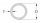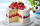# Marbles

Dave had 40 marbles. Junjun has 2 1/5 more than Dave’s marbles. How many marbles do they have altogether?

x =  128

### Step-by-step explanation:Did you find an error or inaccuracy? Feel free to write us. Thank you!Tips to related online calculators
Need help to calculate sum, simplify or multiply fractions? Try our fraction calculator.

## Related math problems and questions:

• Fractions and mixed numerals(a) Convert the following mixed numbers to improper fractions. i. 3 5/8 ii. 7 7/6 (b) Convert the following improper fraction to a mixed number. i. 13/4 ii. 78/5 (c) Simplify these fractions to their lowest terms. i. 36/42 ii. 27/45 2. evaluate the follow
• Bubble bee marblesMark has 100 marbles. Seventeen are bubble bee marbles. What decimal number shows the fraction of marbles that are bumblebees?
• Area and perimeter 2Find the area and the perimeter of a rectangle of length 45 1/2 cm and breadth 16 2/3 cm.
• How many 14How many 1/2 cup serving are in a package of cheese that contains 5 1/4 cups altogether?
• TimeWrite time in minutes rounded to one decimal place: 3 h 48 m 45 s.
• Divide 11Divide the product of 4 and 5/8 by 1 1/2. Write your answer as a mixed number.3 3/4 + 2 3/5 + 5 1/2 Show your solution.
• Eight pipesEight pipes are each 2¼m long. What is the total length of the eight pipes?
• BarrelsPeter has 42 barrels. One-sixth of them are filled with lemonade, one third is filled with wine and half is empty. Calculate how many barrels are with each filling.
• Evaluate mixed expressionsWhich of the following is equal to 4 and 2 over 3 divided by 3 and 1 over 2? A. 4 and 2 over 3 times 3 and 2 over 1 B. 14 over 3 times 2 over 7 C. 14 over 3 times 7 over 2 D. 42 over 3 times 2 over 31Add two mixed fractions: 2 4/6 + 1 3/6Evaluate 2x+6y when x=- 4/5 and y=1/3. Write your answer as a fraction or mixed number in simplest form.Lucy and JoJo need to make 140 cupcakes for the school dance. Lucy made 3/5 of the cupcakes. JoJo made 42 cupcakes. What fraction of the cupcakes do Lucy and JoJo still need to make?When (7¹/3 + 2¾)÷(2 + 2¼ × 1¹/3) is simplified the result is?Forty five of the 80 students were girls. What is the ratio of girls to boys?With what number should be divided mixed number 2 3/4 to get 11/12?The sum of 3 mixed numbers is 20 13/15. two of the numbers are 6 1/3 and 7 5/6. what is the third number?